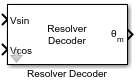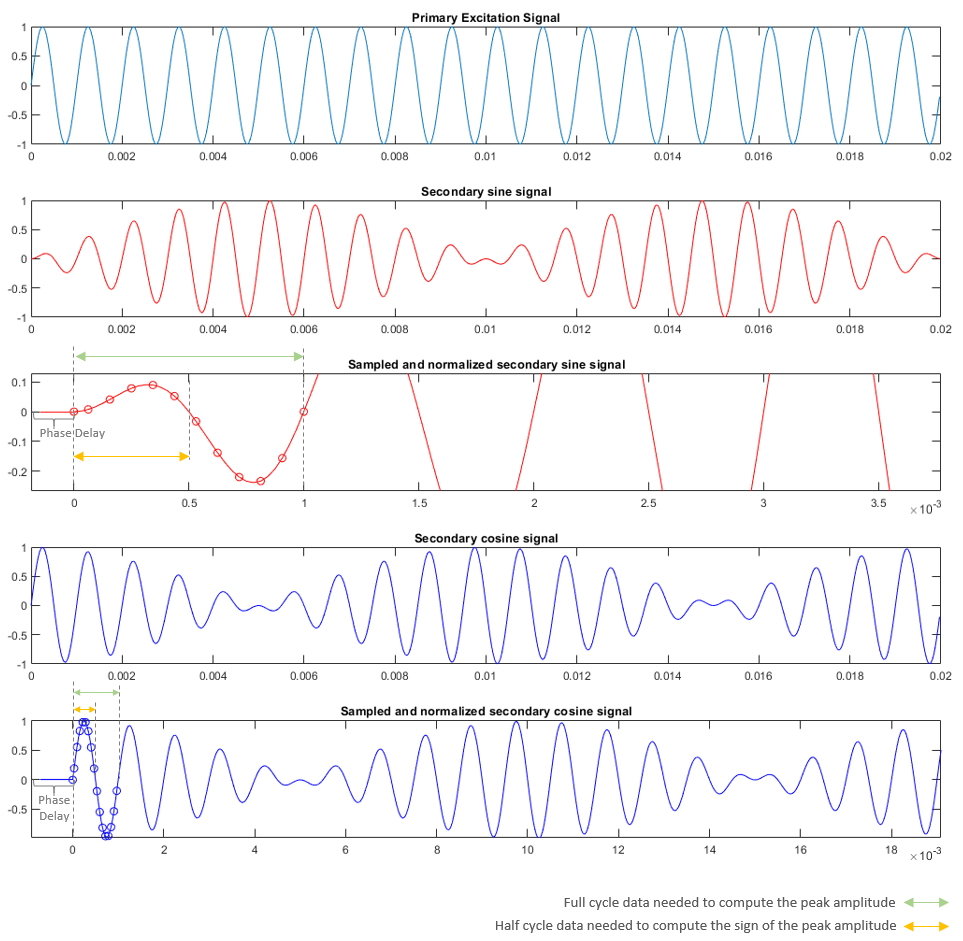# Resolver Decoder

Compute electrical angular position of resolver

• Library:
• Motor Control Blockset / Sensor Decoders

•## Description

The Resolver Decoder block calculates the electrical angular position of the resolver from the resolver sine and cosine output signals.

The resolver uses a primary sinusoidal excitation input signal to generate the modulated secondary sine and cosine waveforms.

You must normalize these waveforms (within the range of [-1,1] and centered at 0) and sample them to obtain the secondary sine and cosine input signals of the Resolver Decoder block.

The block computes and outputs the resolver position in [0, 2π] radians. The block can also add a phase delay to the sampled sine and cosine signals with respect to the excitation signal.Note

The block inputs should have identical amplitude and data types (either signed fixed or floating point).

### Equations

The block computes the average, peak amplitude values, and the sign of the peak amplitude of a signal cycle as

`${Å}_{average}=\frac{1}{n}\sum _{i=0}^{n-1}\left(|{Å}_{i}|\right)$`
`${Å}_{peak}={Å}_{average}×\frac{\pi }{2}$`

where:

• ${Å}_{average}$ is the average amplitude value of a signal cycle

• $n$ is the number of samples per excitation cycle

• ${Å}_{peak}$ is the peak amplitude value of a signal cycle

The block computes the electrical angular position of the resolver as

where:

• ${u}_{\text{sin}_peak}$ is the ${Å}_{peak}$ of the secondary sine signal

• ${u}_{\text{cos}_peak}$ is the ${Å}_{peak}$ of the secondary cosine signal

• $\theta$ is the electrical angular position of the resolver

## Ports

### Input

expand all

Secondary sine waveform output from the resolver that is sampled and normalized within the range of [-1, 1] and centered at 0.

Data Types: `single` | `double` | `fixed point`

Secondary cosine waveform output from the resolver that is sampled and normalized within the range of [-1, 1] and centered at 0.

Data Types: `single` | `double` | `fixed point`

### Output

expand all

Electrical angular position of the resolver (and the rotor) in [0, 2π] radians.

Data Types: `single` | `double` | `fixed point`

## Parameters

expand all

The phase delay that the block must add to the `Sin` and `Cos` input port signals.

Number of samples available in one cycle of the `Sin` and `Cos` input port signals.

The data type of the resolver position output `θ`.